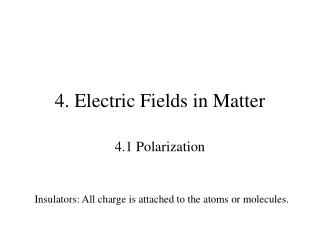DownloadDownload Presentation4. Electric Fields in Matter

# 4. Electric Fields in Matter

Download Presentation## 4. Electric Fields in Matter

- - - - - - - - - - - - - - - - - - - - - - - - - - - E N D - - - - - - - - - - - - - - - - - - - - - - - - - - -
##### Presentation Transcript

1. 4. Electric Fields in Matter 4.1 Polarization Insulators: All charge is attached to the atoms or molecules.

2. (v - Volume) Point charge in a homogenously charged sphere

3. Molecules

4. Polar molecules have a permanent dipole.

5. Take a small volume v that contains, say, N=1000 atoms. Potential generated by the microscopic dipoles: 4.2 The Field of a Polarized Object Polarization P (dipole moment per unit volume) tells how strongly the atoms/molecules are polarized and/or aligned with the electric field.

6. volume charge density surface charge density Bound charges

7. Example 4.2 Electric field of a uniformly polarized sphere.

8. constant field dipole at the center of the sphere

9. The field inside a dielectric Deriving the expressions for the bound charges we considered pure dipoles. The real dielectric contains physical dipoles. The electric field is much more complicated near the molecular dipoles. The macroscopic field is the average over a small volume that contains many molecules. The average field of the pure and molecular dipoles is the same.

10. Total charge Free charge (at our disposal) Bound charge (induced, comes along) Electric displacement (auxiliary field) But in general Boundary conditions 4.3 The Electric Displacement

11. Example 4.4 Long straight wire with uniform line charge is surrounded by a rubber insulation. Find the electric displacement.

12. linear dielectric electric susceptibility permittivity permittivity of free space relative permittivity, dielectric constant 4.4 Linear Dielectrics Most macroscopic fields are weak as compared to the atomic and molecular fields. The polarization is weak.

13. Dielectric constants Table

14. a) When the space is filled with a homogenous dielectric. On may calculate D in the same way as E in the vacuum if the different boundary conditions for E and D do not play role. In this case, one simply replaces This is the case if: b) When the symmetry of the problem makes

15. Charge embedded in a homogenous dielectric material. Bound charges partially screen q.

16. D dielectric D=0 Air: dielectric constant dielectric strength Parallel plate capacitor filled with a dielectric. • Dielectrics are used to: • Increase the capacity • Keep the plates apart • Increase the dielectric strength (field strength without a spark)

17. A cut section of a multiplayer capacitor with a ceramic dielectric. Ceramic capacitors

18. Foil wound capacitor. Frequently used dielectrics: Paper Mica Polysterene

19. Example 4.5 Metal sphere of radius a carries a charge Q. It is surrounded by a linear dielectric material. Find the potential at the center.

20. Displacement at a boundary without free charge.

21. Within a homogenous linear dielectric, Laplace’s equation holds. Boundary conditions on the surface between two dielectrics: 4.4 Boundary Problems with Linear Dielectrics

22. Example 4.7 A sphere of homogeneous dielectric material is placed into an otherwise uniform electric field. Find the field inside the sphere.

23. Example 4.8 Find the electrical field inside and outside the dielectric and the force on the charge.

24. For linear dielectrics 4.5 Energy in a dielectric system Capacitor

25. Force on a dielectric